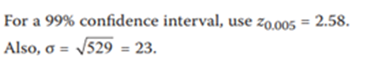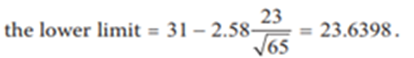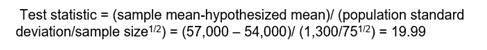# Buổi 3 - Quantitative - Reading 10: Sampling and Estimation & Reading 11: Hypothesis Testing

READING 10: SAMPLING AND ESTIMATION

Question 10.1: Thomas Merton, a car industry analyst, wants to investigate a relationship between the types of ads used in advertising campaigns and sales to customers in certain age groups. In order to make sure he includes manufacturers of all sizes, Merton divides the industry into four size groups and draws random samples from each group. What sampling method is Merton using?
A) Stratified random sampling.
B) Simple random sampling.
C) Cross-sectional sampling
Explanation
A is correct. In stratified random sampling, we first divide the population into subgroups based on some relevant characteristic(s) and then make random draws from each group.

Question 10.2:
Alan Barnes, CFA, is interested in the expected return on the FTSE 100 stock index next quarter. He has data for the last five years and calculates the average return on the index over the last 20 quarters. This average return:
A) is different from the parameter he is trying to estimate by the amount of the sampling error. B) overstates the return because he should divide by the square root of 20 when using a mean value.
C) overstates the expected return because he should have used the geometric mean and not the simple average.
Explanation
A is correct. The 20 quarters he has used are a sample of all the possible outcomes for the quarterly returns on the index. The difference between the true population parameter (mean index return) he is trying to estimate and the sample statistic he has calculated is called the sampling error. The arithmetic mean is the appropriate estimator of the next period's return.

Question 10.3: An analyst is asked to calculate standard deviation using monthly returns over the last five years. These data are best described as:
A) cross-sectional data.
B) systematic sampling data.
C) time series data
Explanation
C is correct. Time series data are taken at equally spaced intervals, such as monthly, quarterly, or annual. Cross sectional data are taken at a single point in time. An example of cross-sectional data is dividend yields on 500 stocks as of the end of a year.

Question10.4: Which of the following statements about sample statistics is least accurate?
A) The z-statistic is used for non normal distributions with known variance, but only for large samples.
B) There is no sample statistic for non-normal distributions with unknown variance for either small or large samples.
C) The z-statistic is used to test normally distributed data with a known variance, whether testing a large or a small sample.
Explanation
B is correct. There is no sample statistic for non-normal distributions with unknown variance for small samples, but the t-statistic is used when the sample size is large.

Question 10.5: An increase in sample size is most likely to result in a :
A wider confidence interval.
B decrease in the standard error of the sample mean.
C lower likelihood of sampling from more than one population.
Explanation
B is correct. All else being equal, as the sample size increases, the standard error of the sample mean decreases and the width of the confidence interval also decreases.

Question 10.6:

Which of the following statements about the central limit theorem is least accurate?

A) The central limit theorem has limited usefulness for skewed distributions.
B) The mean of the population and the mean of all possible sample means are equal.
C) When the sample size is large, the sampling distribution of the sample means is approximately normal.

Explanation
A is correct. The central limit theorem holds for any distribution as long as the sample size is large (i.e., n > 30)

Question 10.7:
A traffic engineer is trying to measure the effects of carpool-only lanes on the expressway. Based on a sample of 100 cars at rush hour, he finds that the mean number of occupants per car is 2.5, and the sample standard deviation is 0.4. What is the standard error of the sample mean?
A) 1.00.
B) 5.68.
C) 0.04.
Explanation
C is correct. The standard error of the sample mean when the standard deviation of the population is not known is estimated by the standard deviation of the sample divided by the square root of the sample size = 0

Question 10.8: The sample mean is a consistent estimator of the population mean because the:
A) sampling distribution of the sample mean has the smallest variance of any other unbiased estimators of the population mean.
B) sample mean provides a more accurate estimate of the population mean as the sample size increases.
C) expected value of the sample mean is equal to the population mean.

Explanation

B is correct. A consistent estimator provides a more accurate estimate of the parameter as the sample size increases.

Question 10.9: For a sample size of 65 with a mean of 31 taken from a normally distributed population with a variance of 529, a 99% confidence interval for the population mean will have a lower limit closest to:
A) 23.64.
B) 25.41.
C) 30.09.
Explanation
Confidence interval = Point estimate +/- reliability factor x standard error
Step 1: Determine each factor
Known population variance => z distribution can be usedStep 2: Calculate the lower limitQuestion 10.10:

Compared to a t-distribution with 10 degrees of freedom, and compared to a normal distribution, a t-distribution with 20 degrees of freedom and the same variance has:

Compared to df = 10     Compared to normal

A)        thinner tails                    flatter tails
B)       flatter tails                      thinner tails
C)       flatter tails                      flatter tails

Explanation

A is correct. A t-distribution with sufficiently high degrees of freedom is approximately normal and a normal distribution has thinner tails compared to a t-distribution. The less the degrees of freedom, the flatter the tails.

Question 10.11: A study reports that from 2002 to 2004 the average return on growth stocks was twice as large as that of value stocks. These results most likely reect:
B) time-period bias.
C) survivorship bias.
Explanation
B is correct. Time-period bias can result if the time period over which the data is gathered is either too short because the results may reflect phenomenon specific to that time period, or if a change occurred during the time frame that would result in two different return distributions. In this case the time period sampled is probably not large enough to draw any conclusions about the long-term relative performance of value and growth stocks, even if the sample size within that time period is large.

READING 11: HYPOTHESIS TESTING

Question 11.1: In order to test whether the mean IQ of employees in an organization is greater than 100, a sample of 30 employees is taken and the sample value of the computed test statistic, tn-1 = 3.4. The null and alternative hypotheses are:
A) H0: X ≤ 100; Ha: X > 100.
B) H0: µ ≤ 100; Ha: µ > 100.
C) H0: µ = 100; Ha: µ ≠ 100.
Explanation
B is correct. The null hypothesis is that the population mean is less than or equal to from 100. The alternative hypothesis is that the population mean is greater than 100.

Question 11.2: The level of significance of a hypothesis test is best used to:
A calculate the test statistic.
B define the test’s rejection points.
C specify the probability of a Type II error.
Explanation
B is correct. The level of significance is used to establish the rejection points of the hypothesis test.

Question 11.3:
Which of the following statements about hypothesis testing is least accurate?
A) A Type I error is the probability of rejecting the null hypothesis when the null hypothesis is false.
B) A Type II error is the probability of failing to reject a null hypothesis that is not true.
C) The significance level is the probability of making a Type I error.
Explanation
A is correct. A Type I error is the probability of rejecting the null hypothesis when the null hypothesis is true.

Question 11.4: An analyst tests the profitability of a trading strategy with the null hypothesis being that the average abnormal return before trading costs equals zero. The calculated t -statistic is 2.802, with critical values of ± 2.756 at significance level α = 0.01. After considering trading costs, the strategy’s return is near zero. The results are most likely:
A. statistically but not economically significant.
B. economically but not statistically significant.
C. neither statistically nor economically significant.
Explanation
A is correct. The hypothesis is a two-tailed formulation. The t -statistic of 2.802 falls outside the critical rejection points of less than –2.756 and greater than 2.756, therefore the null hypothesis is rejected; the result is statistically significant. However, despite the statistical results, trying to profit on the strategy is not likely to be economically meaningful because the return is near zero after transaction costs.

Question 11.5: A survey is taken to determine whether the average starting salaries of CFA charter holders is equal to or greater than \$54,000 per year. Assuming a normal distribution, what is the test statistic given a sample of 75 newly acquired CFA charter holders with a mean starting salary of \$57,000 and a standard deviation of \$1,300?
A) 19.99.
B) 2.31.
C) -19.99
Explanation
A large sample size and unknown population standard deviation unknown => Z distribution can be usedQuestion 11.6: For a test with sample size n of whether two variables are correlated, the critical values are based on:
A) n degrees of freedom.
B) n – 1 degrees of freedom.
C) n – 2 degrees of freedom.
Explanation
C is correct. The test statistic for the hypothesis that correlation = 0 follows a t-distribution with n – 2 degrees of freedom.

Question 11.7: Joe Bay, CFA, wants to test the hypothesis that the variance of returns on energy stocks is equal to the variance of returns on transportation stocks. Bay assumes the samples are independent and the returns are normally distributed. The appropriate test statistic for this hypothesis is:
A) a Chi-square statistic.
B) F-statistic.
C) a t-statistic.
Explanation
B is correct. Bay is testing a hypothesis about the equality of variances of two normally distributed populations. The test statistic used to test this hypothesis is an F-statistic. A chi-square statistic is used to test a hypothesis about the variance of a single population. A t-statistic is used to test hypotheses concerning a population mean.

Question 11.8: If a one-tailed z-test uses a 5% significance level, the test will reject a:
A) true null hypothesis 5% of the time.
B) false null hypothesis 95% of the time.
C) true null hypothesis 95% of the time.
Explanation
A is correct. The level of significance is the probability of rejecting the null hypothesis when it is true. The probability of rejecting the null when it is false is the power of a test.

Question 11.9: An analyst is examining the monthly returns for two funds over one year. Both funds’ returns are non-normally distributed. To test whether the mean return of one fund is greater than the mean return of the other fund, the analyst can use:
A) a parametric test only.
B) a nonparametric test only.
C) both parametric and nonparametric tests
Explanation
B is correct. There are only 12 (monthly) observations over the one year of the sample and thus the samples are small. Additionally, the funds’ returns are non-normally distributed. Therefore, the samples do not meet the distributional assumptions for a parametric test. The Mann–Whitney U test (a nonparametric test) could be used to test the differences between population means.